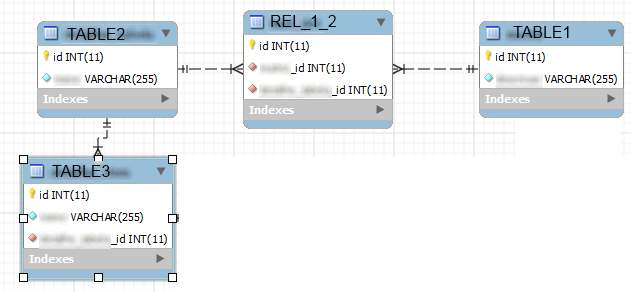# Relation between 3 tables

I have the following problem

3023Table1 has a MANY_MANY relation between Table2. And Table2 has a HAS_MANY relation between Table3. Everything is good inside model classes.

Inside my view, I could do:

``````
\$table1 = Table1::model()->with('table2')->findByPk(\$pk);

``````

And get all the table2 data that is associated with that Table1 row using

``````
\$table1->table2;

``````

But, if I wanted to get all Table3 data, I would do:

``````

\$table1 = Table1::model()->with('table2')->findByPk(\$pk);

\$table2 = \$table1->table2;

\$table2Ids = array();

foreach (\$table2 as \$t) {

array_push(\$table2Ids , \$t['id']);

}

\$table2 = Table2::model()->with('table3')->findByPk(\$table2Ids);

\$table3 = \$table2->table3;

``````

Is there another way to do this? Something like :

``````

Table1::model()->with('table2')->with('table3')->findByPk(\$pk)->table2->table3;

``````
``````

Table1::model()->with('table2')->with('table2.table3')->findByPk(\$pk);

``````

try this

``````
\$table1 = Table1::model()->with(array('table2'=>array('with'=>'table3')))->findByPk(\$pk);

``````

Redguy and Rudenich, thank you for the replies. I tried those two, none of them seems to work. Maybe I misunderstood something because the name of the relations are the name of the tables. So rel_table2 & rel_table3 are the strings defined inside each models public function relations().

``````
Table1::model()->with(array('rel_table2'=>array('with'=>'rel_table3')))->findByPk(\$pk)->rel_table2->rel_table3

``````

Gives me a "Trying to get property of non-object".

``````
Table1::model()->with('rel_table2')->with('rel_table2.rel_table3')->findByPk(\$pk)->rel_table2->rel_table3

``````

Gives me a "Trying to get property of non-object".

Using my code mentioned before gives me the right results. Am I doing something wrong?

I think previous provided examples should work (customized according to your exact needs).

Don’t have enough time to properly test your case, but here’s a couple of checks I’d first do:

1. First of all, I’d define what exactly returns Trying to get property of non-object, var-dumping results of
``````
\$table1 = Table1::model()->with('rel_table2.rel_table3')->findByPk(\$pk)

``````
``````
\$table1->rel_table2

``````
1. Then I’d check rendered sql - if it looks proper way gives an expected result

2. There’s a possibility you have NULL result for one of your joins, so maybe this is the case

I tried the following:

``````
\$table1 = Table1::model()->with('rel_table2.rel_table3')->findByPk(\$pk);

``````

This works and brings the correct data;

Then, I do

``````
\$table1->rel_table2;

``````

Works too;

But when I try to

``````
\$table1->rel_table2->rel_table3;

``````

Brings me the "Trying to get property of non-object".

There is no NULL result because I do the same thing with the same data, just in a different manner and it works (see 1st post).

I enabled CWebLogRoute and, for my surprise, the SQL is correct. I copy/paste inside the database and got the correct results. This system.db.CDbCommand is running on this line of code:

``````
\$table1 = Table1::model()->with('rel_table2.rel_table3')->findByPk(\$pk);

``````

So, this must be correct.

But why \$table1->rel_table2->rel_table3; is giving me the "Trying to get property of non-object" error?

Notes:

Table1 is has:

``````
public function behaviors() {

}

``````

AND

``````
public function relations() {

return array(

'rel_table2' => array(self::MANY_MANY, 'Table2', 'rel_1_2(table1_id, table2_id)'),

);

}

``````

Table2 has:

``````
public function relations() {

return array(

'rel_table3' => array(self::HAS_MANY, 'Table3', 'table2_id'),

'rel_table1' => array(self::MANY_MANY, 'Table1', 'rel_1_2(table2_id, table1_id)'),

);

}

``````